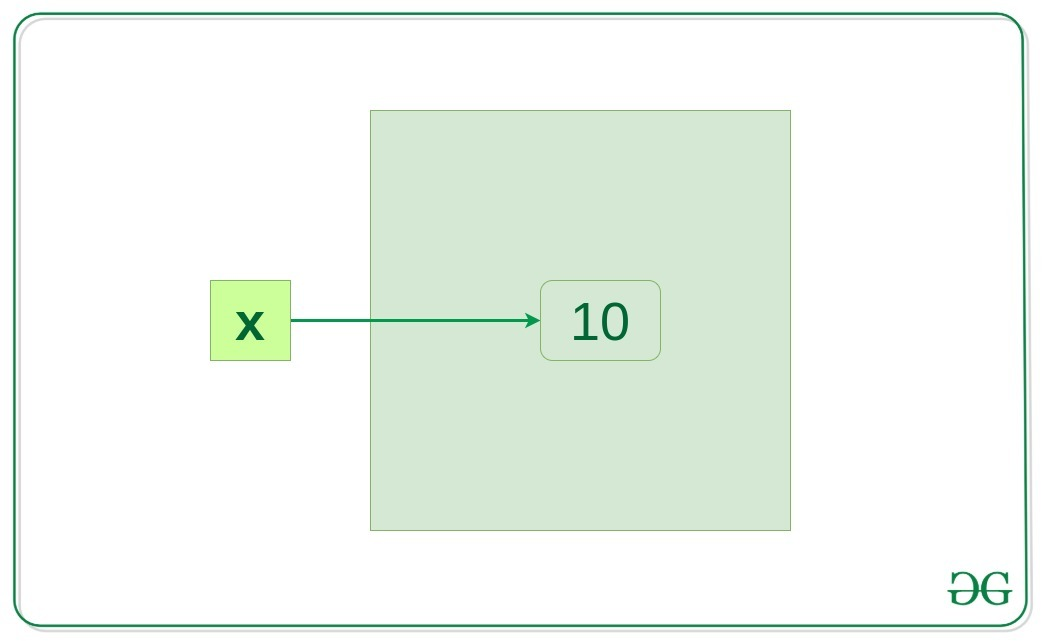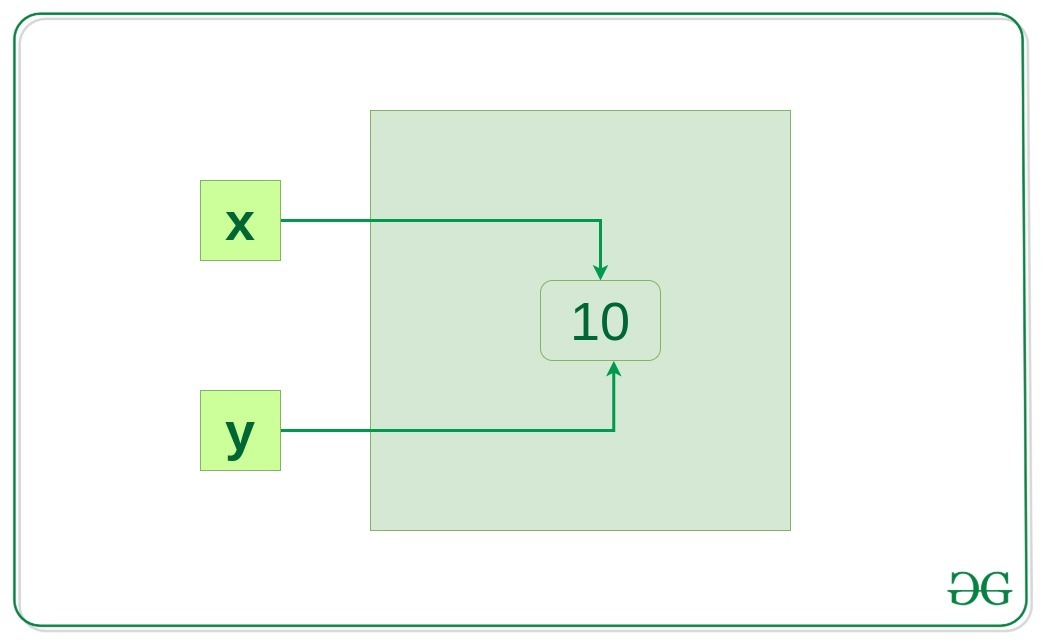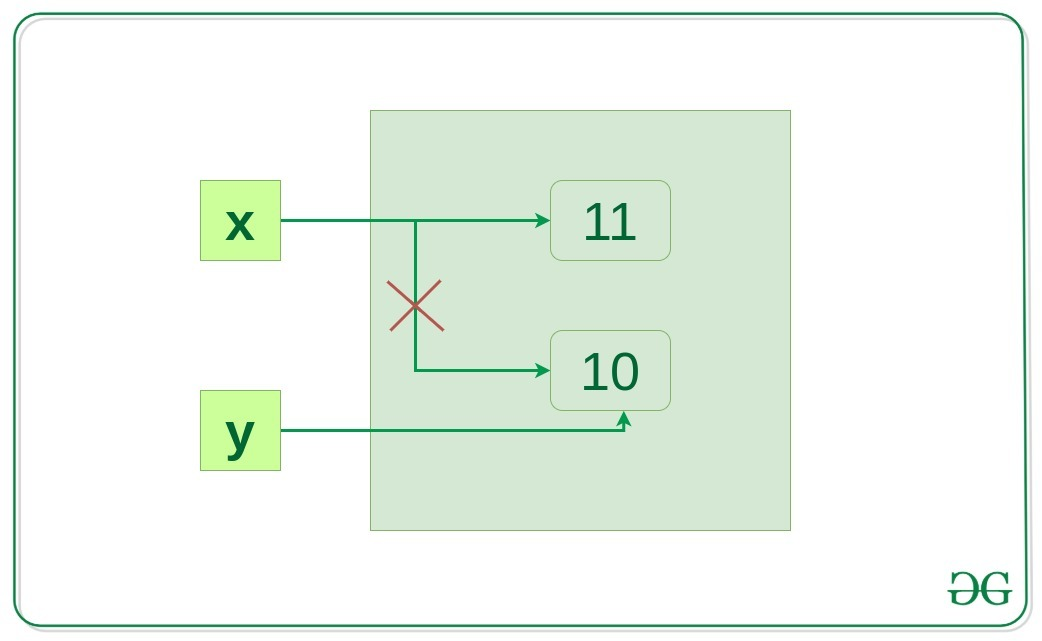# Memory Management in Python

• Difficulty Level : Easy
• Last Updated : 10 May, 2020

Understanding Memory allocation is important to any software developer as writing efficient code means writing a memory-efficient code. Memory allocation can be defined as allocating a block of space in the computer memory to a program. In Python memory allocation and deallocation method is automatic as the Python developers created a garbage collector for Python so that the user does not have to do manual garbage collection.

## Garbage Collection

Garbage collection is a process in which the interpreter frees up the memory when not in use to make it available for other objects.
Assume a case where no reference is pointing to an object in memory i.e. it is not in use so, the virtual machine has a garbage collector that automatically deletes that object from the heap memory

## Reference Counting

Reference counting works by counting the number of times an object is referenced by other objects in the system. When references to an object are removed, the reference count for an object is decremented. When the reference count becomes zero, the object is deallocated.

For example, Let’s suppose there are two or more variables that have the same value, so, what Python virtual machine does is, rather than creating another object of the same value in the private heap, it actually makes the second variable point to that originally existing value in the private heap. Therefore, in the case of classes, having a number of references may occupy a large amount of space in the memory, in such a case referencing counting is highly beneficial to preserve the memory to be available for other objects

Example:

 `x ``=` `10`

When `x = 10` is executed an integer object 10 is created in memory and its reference is assigned to variable x, this is because everything is object in Python.Let’s verify if it’s true

 `x ``=` `10``y ``=` `x`` ` `if` `id``(x) ``=``=` `id``(y):``    ``print``(``"x and y refer to the same object"``)`

Output:

`x and y refer to the same object`

In the above example, `y = x` will create another reference variable y which will refer to the same object because Python optimizes memory utilization by allocation the same object reference to a new variable if the object already exists with the same value.Now, let’s change the value of x and see what happens.

 `x ``=` `10``y ``=` `x``x ``+``=` `1`` ` `if` `id``(x) !``=` `id``(y):``    ``print``(``"x and y do not refer to the same object"``)`

Output:

`x and y do not refer to the same object`

So now x refer to a new object x and the link between x and 10 disconnected but y still refer to 10.## Memory Allocation in Python

There are two parts of memory:

• stack memory
• heap memory

The methods/method calls and the references are stored in stack memory and all the values objects are stored in a private heap.

### Work of Stack Memory

The allocation happens on contiguous blocks of memory. We call it stack memory allocation because the allocation happens in the function call stack. The size of memory to be allocated is known to the compiler and whenever a function is called, its variables get memory allocated on the stack.

It is the memory that is only needed inside a particular function or method call. When a function is called, it is added onto the program’s call stack. Any local memory assignments such as variable initializations inside the particular functions are stored temporarily on the function call stack, where it is deleted once the function returns, and the call stack moves on to the next task. This allocation onto a contiguous block of memory is handled by the compiler using predefined routines, and developers do not need to worry about it.

Example:

 `def` `func(): ``       ` `    ``# All these variables get memory  ``    ``# allocated on stack  ``    ``a ``=` `20``    ``b ``=` `[] ``    ``c ``=` `"" `

### Work of Heap Memory

The memory is allocated during the execution of instructions written by programmers. Note that the name heap has nothing to do with the heap data structure. It is called heap because it is a pile of memory space available to programmers to allocated and de-allocate. The variables are needed outside of method or function calls or are shared within multiple functions globally are stored in Heap memory.

Example:

 `# This memory for 10 integers  ``# is allocated on heap.  ``a ``=` `[``0``]``*``10`

My Personal Notes arrow_drop_up